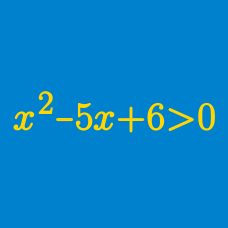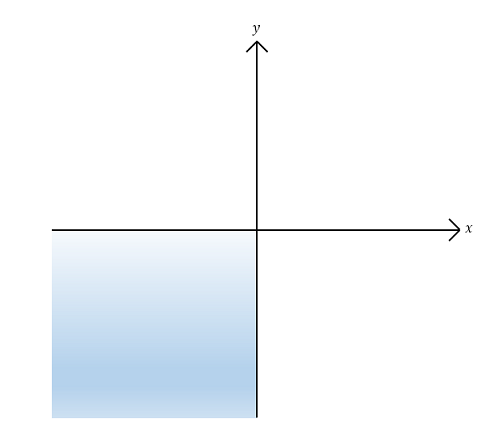Algebra

# Polynomial Inequalities - Sets

$A$ and $B$ are two subsets of $\mathbb{R}$ defined by: \begin{aligned} A &= \{ x \mid x^2 - 6 x - 91 \leq 0 \}, \\ B &= \{ x \mid x^2 - 6 x - 91 = 0 \}. \end{aligned} How many integers does the set $A \cap B^C$ contain?

Details and assumptions

$B^C$ denotes the complement of set $B$.

$\mathbb{R}$ denotes the set of all real numbers.Sets $A, B, C$ and $D$ are defined as follows: \begin{aligned} A &= \{ (x,y) \vert x>0 \} \\ B &= \{ (x,y) \vert y>0 \} \\ C &= \{ (x,y) \vert y>5x \} \\ D &= \{ (x,y) \vert y<-3x \}. \end{aligned} Then which of the following is the set that represents the shaded region, including the two axes, in the above graph?

The sets $A = \{ x \mid x^2 - 5 x + 4 \leq 0 \}$ and $B = \{ x \mid x^2 + ax + b < 0 \}$ satisfy the following conditions: $A \cap B = \emptyset \mbox{ and } A \cup B = \{ x \mid 1 \leq x < 15 \}.$ What is the value of $a + b?$

$P,\ Q$ and $R$ are three subsets of $\mathbb{R}$ defined as follows: \begin{aligned} P &= \{ x \mid x^2 - 21x + 110 > 0 \}, \\ Q &= \{ x \mid x^2 - 15x + 44 < 0 \}, \\ R &= \{ x \mid x^2 - 11ax + 10a^2 < 0,\ a > 0 \}. \end{aligned} What is the sum of the minimum and maximum values of $a$ such that $(P \cap Q) \subset R$?

Details and assumptions

$\mathbb{R}$ denotes the set of all real numbers.

$A$, $B$ and $C$ are subsets of $\mathbb{R}$ defined as follows: \begin{aligned} A &= \{ x \mid x^2 - 10 x + 21 \geq 0 \}, \\ B &= \{ x \mid x^2 - 10 x + 21 > 0 \},\\ C &= \{ x \mid x^2 - 19 x + 48 = 0 \}. \end{aligned} What is the value of the sum of all the elements of the set $(A \cap B^C) \cup C$?

Details and assumptions

$B^C$ denotes the complement of set $B$.

$\mathbb{R}$ denotes the set of all real numbers.

×